# Converting Text to Its Proper Case

In this article, we will learn how to use PROPER function in Microsoft Excel.

In Excel, PROPER function is used to capitalize the first letter of every word in a given string. That means, all letters that do not follow any other letter are set to uppercase and the rest to lowercase. The function is very useful to change the case irregularities to make the data look good.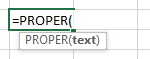Let’s take examples and understand:-

Here, we have taken few random text values in column A. And in column B, we will enter the function to return the output.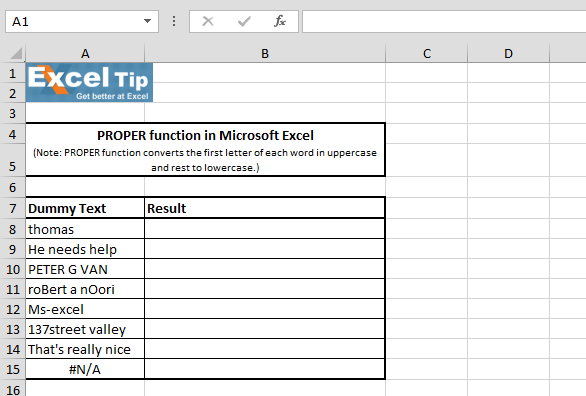1st Example:-

In this example, we will convert the text form lower case to proper case.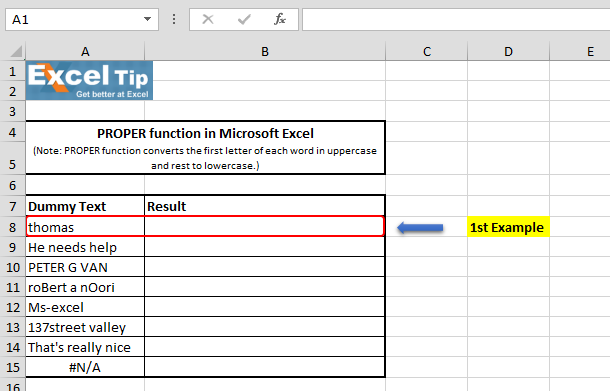Follow the steps given below:-

• Enter the function in cell B8
• =PROPER(A8), Press Enter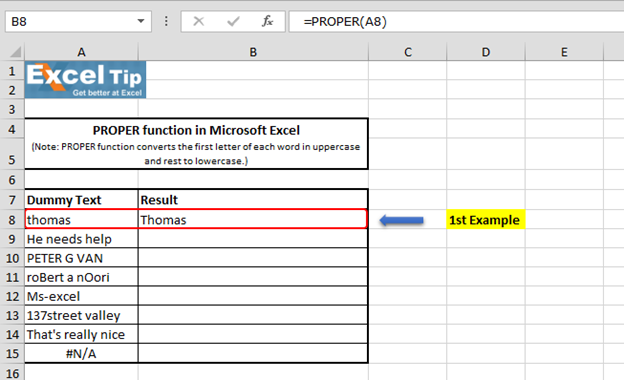The function has capitalized the first letter of the string which is letter “T” and returned.

2nd Example:-

In this example, in a cell we have 3 text strings in which only first letter is in capital and the rest in small.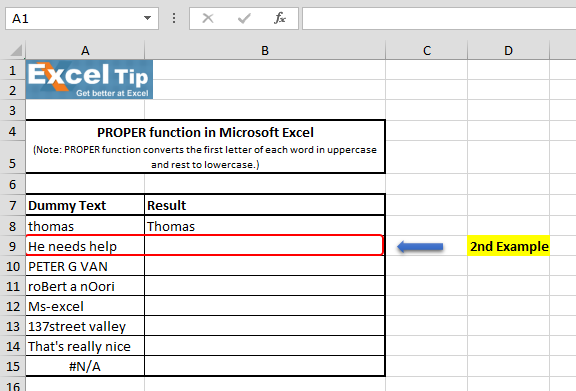Follow the steps given below:-

• Enter the function in cell B9
• =PROPER(A9), Press Enter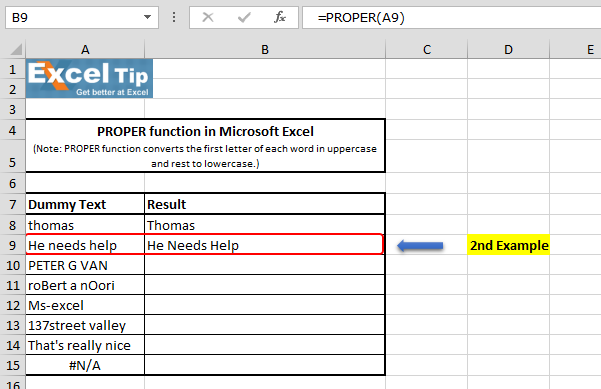PROPER function capitalizes the first letter of each word and returned.

3rd Example:-

In this example, we have all the letters in capital. Let’s see how PROPER function will perform?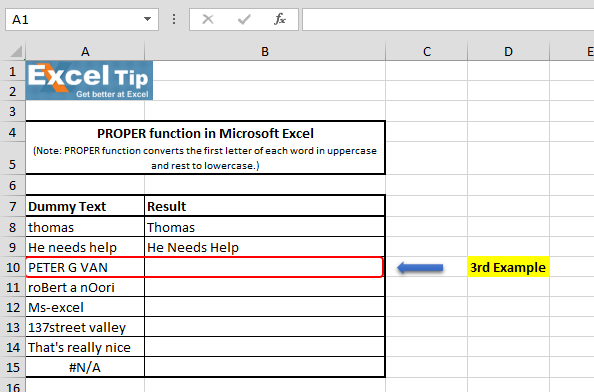Follow the steps given below:-

• Enter the function in cell B10
• =PROPER(A10), Press Enter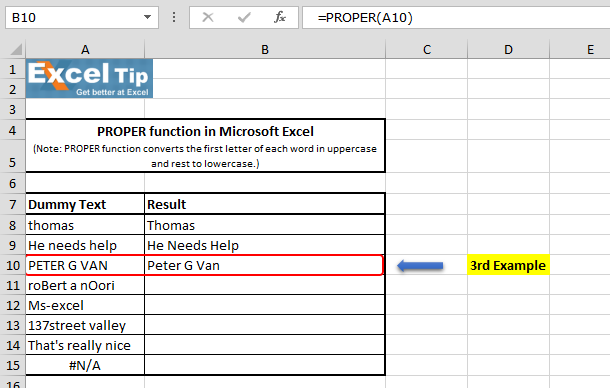The Function has returned “Peter G Van” as an answer, where the “P” “G” & “V” are still capital and remaining characters have changed to lowercase. It is because, function analyzed each word in the string and kept the first character in each word as it is. And, all remaining characters converted to lowercase.

4th Example:-

In this example, we will see what happens if a dreadful muddle text case is supplied in the argument?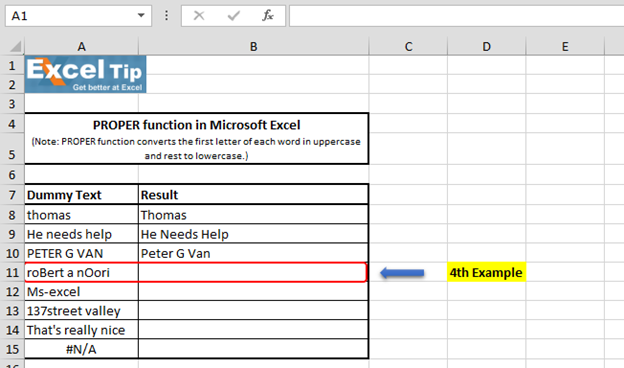Follow the steps given below:-

• Enter the function in cell B11
• =PROPER(A11), Press Enter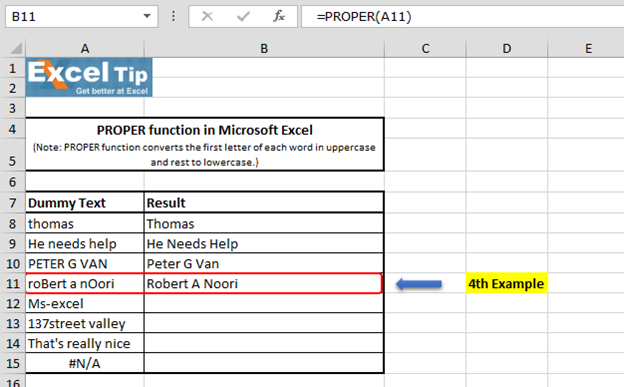The function has returned the answer with capitalizing the first letter of each word which looks more readable as compared to the original string.

5th Example:-

In this example, we have taken special character instead of space. Let’s see how the function performs.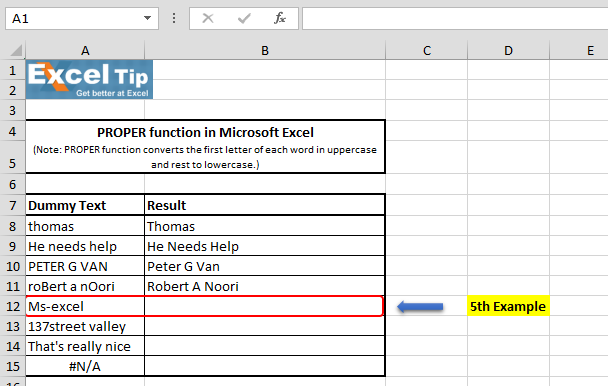Follow the steps given below:-

• Enter the function in cell B12
• =PROPER(A12), Press Enter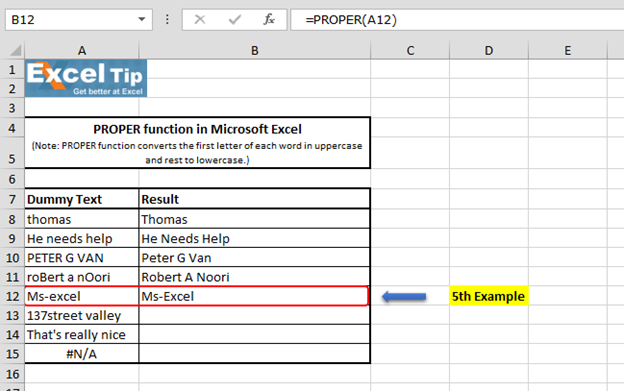Function has changed “M”, “E” in upper case and rest in lower case. It is because function only affects letters that do not follow any other letter. Numbers and punctuation characters are not affected. And, here as letter “E” is not followed by any other letter; therefore, it is converted to uppercase.

6th Example:-

In this example, we have taken numeric value at the starting place. Let’s see how the function will perform now?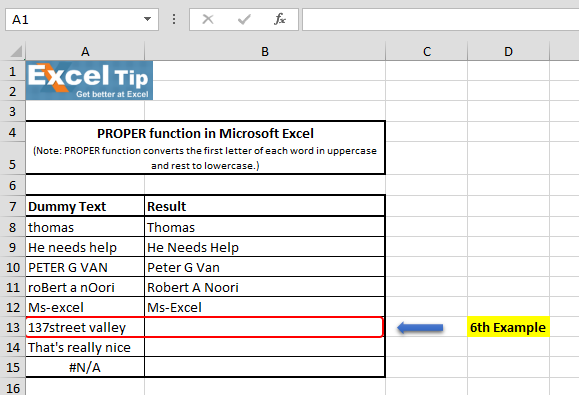Follow the steps given below:-

• Enter the function in cell B13
• =PROPER(A13), Press Enter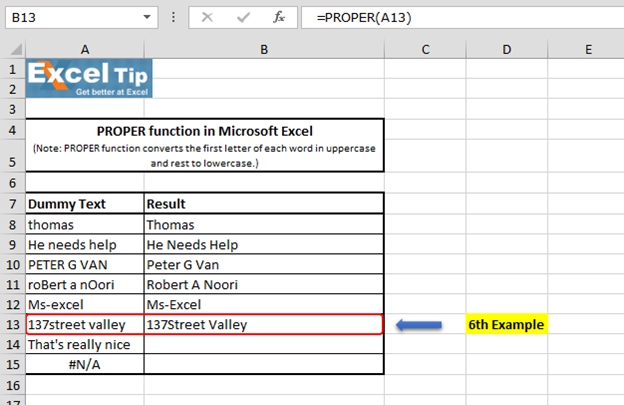The function has returned and converted the first letter “S” which is after the number “7” to uppercase and then, it converts the letter “S” which is the first letter of the next word.

7th Example:-

In this example, let’s see what happens if you have such text in your database.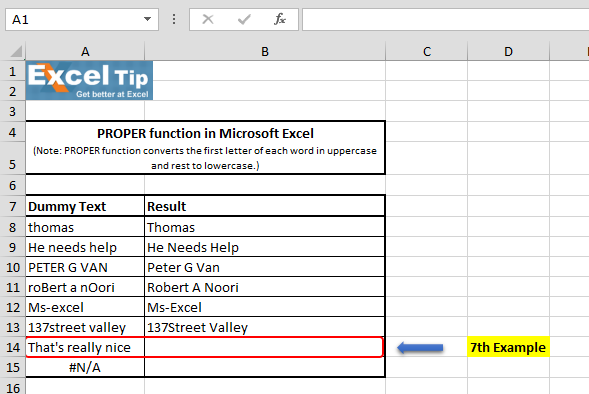Follow the steps given below:-

• Enter the function in cell B14
• =PROPER(A14), Press Enter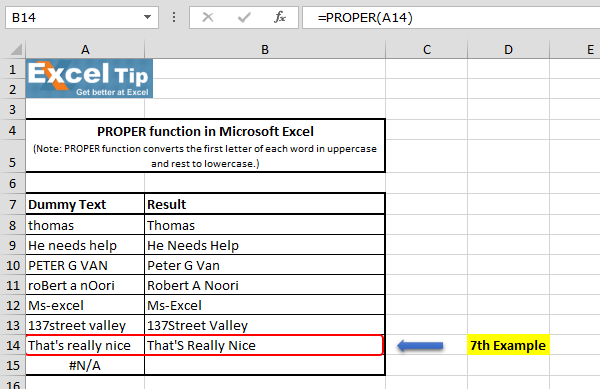In the above example, everything is looks fine except for the letter “S” which is coming after apostrophe and now in uppercase. It is because of special character, function assumed the very next word is “S” and converted into uppercase. This is one of the disadvantages of this function.

8th Example:-

In this example, we have taken error value.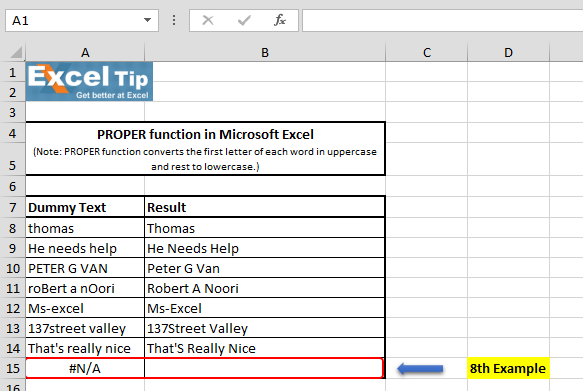Follow the steps given below:-

• Enter the function in cell B15
• =PROPER(A15), Press Enter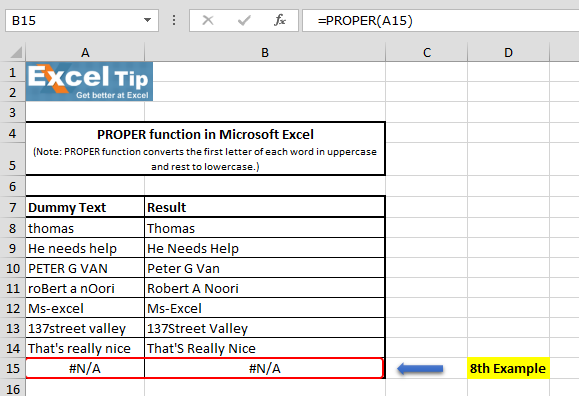Function has returned #N/A error which is there in cell A15. That’s because, function looks only for text value, and in case of any error value, it returns the same error as result.

So, this is how PROPER function works and can be used in different situations in Excel. It is very useful while working on data that contains proper nouns, like names or titles. You can always use the PROPER function to make sure everything is capitalized correctly.# Video: How to use PROPER function in Microsoft Excel

Watch the steps in this short video, and the written instructions are above the video

If you liked our blogs, share it with your friends on Facebook. And also you can follow us on Twitter and Facebook.
We would love to hear from you, do let us know how we can improve, complement or innovate our work and make it better for you. Write us at info@exceltip.com

## Comments

1.pl try to help me

2.YOU CAN USE THE UPPER FORMULA ON THE FIRST CELL, AND THEN USE THE SMALL + AT THE BOTTOM OF THE CELL TO GO TO THE BOTTOM OF THE COLUMN. THIS WILL REPLACE ALL OF THE VALUES IN THE FORMULA WITH THE CELL NUMBERS FOR THE RANGE YOU HIGHLIGHT. THEN JUST REPEAT FOR EACH COLUMN

3."1 if u want column a from 1-10 then copy cell a1 to b1
2 apply upper formula
3 copy formula in b1 to all rows till b10
4 copy b1-b10 and paste-special--> values at position a1"

4."You can down load ASAP Utilites which has a converter to all selected cells.

http://www.asap-utilities.com/"

5.I could not get this to work at all. If any one know a way to convert entire columns to upper case, it would save me a lot of time. thanks.

6.This tip works when converting a single cell, but I cannot find how to convert an entire worksheet like I can in WORD. I tried entering =UPPER(A1:J254) but it did not work. My data list is a chart of names and addresses with columns of figures and I want to change the text parts to upper case.

7.when I enter this formula I get a circular reference. How do I get rid of that. I also would like to convert entire columns at one time. Anyone got the solution.

8.This tip works when converting a single cell, but I cannot find how to convert an entire worksheet like I can in WORD. I tried entering =UPPER(A1:J254) but it did not work. My data list is a chart of names and addresses with columns of figures and I want to change the text parts to upper case.

Terms and Conditions of use

The applications/code on this site are distributed as is and without warranties or liability. In no event shall the owner of the copyrights, or the authors of the applications/code be liable for any loss of profit, any problems or any damage resulting from the use or evaluation of the applications/code.